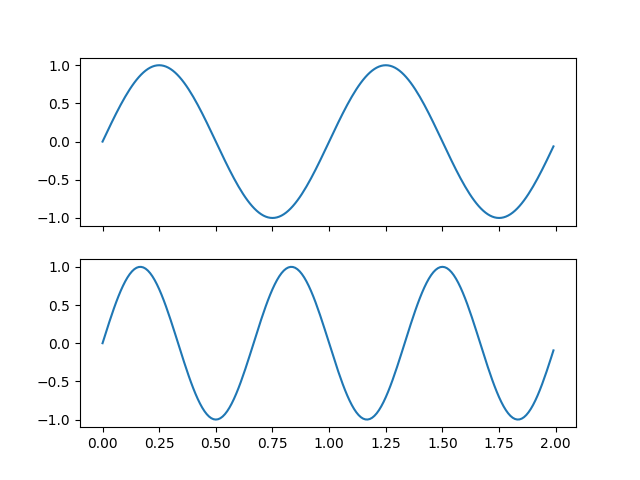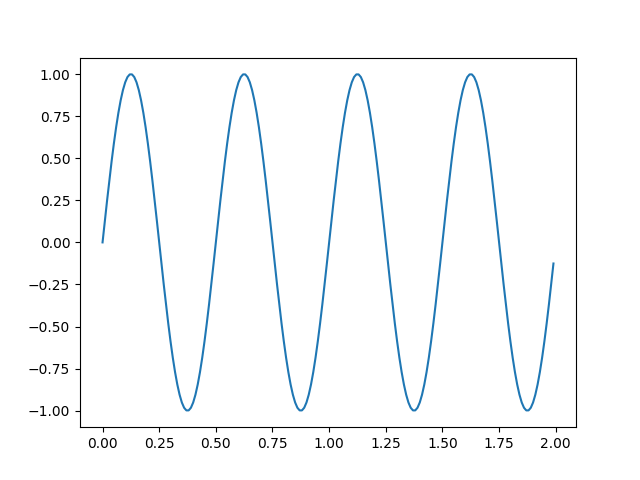# Multicursor#

Showing a cursor on multiple plots simultaneously.

This example generates three axes split over two different figures. On hovering the cursor over data in one subplot, the values of that datapoint are shown in all axes.

import numpy as np
import matplotlib.pyplot as plt
from matplotlib.widgets import MultiCursor

t = np.arange(0.0, 2.0, 0.01)
s1 = np.sin(2*np.pi*t)
s2 = np.sin(3*np.pi*t)
s3 = np.sin(4*np.pi*t)

fig, (ax1, ax2) = plt.subplots(2, sharex=True)
ax1.plot(t, s1)
ax2.plot(t, s2)
fig, ax3 = plt.subplots()
ax3.plot(t, s3)

multi = MultiCursor(None, (ax1, ax2, ax3), color='r', lw=1)
plt.show()

••References

The use of the following functions, methods, classes and modules is shown in this example:

Gallery generated by Sphinx-Gallery